# 乐清湾港区绿荫路建设工程（一期）-乐清湾港区绿荫路建设工程（一期）

2021年06月18   浙江
 所属地区 浙江 中标金额 ****** 相关单位 中标单位： *** 查企业资质、中标业绩> 招标业主： 乐清***公司 查企业资质、中标业绩> 还有336位联系人等你来看哦

﻿

 名次 中标候选人 是否 (略) 企业 投标报价（元） 1 (略) (略) 否 ***

 商务标名次 投标人名称 废标原因 废标依据 (略) 在开标阶段发现 (略) 的投标函工期为 * ，没有日历天。 根据招标文件第 * 页：“2.1 符合性评审标准。开评标时，投标人如存在以下情况之 * 的，作为符合性评审未获通过而予以否决，不得进入后续评审：（7） (略) 文件要求的工程验收、施工工期、保修期等要求的；（8）未按规定的格式、内容填写或不全，关键字迹模糊、无法辨认。” (略) 在开标阶段发现 (略) 的投标函工期为 * ，没有日历天。 根据招标文件第 * 页：“2.1 符合性评审标准。开评标时，投标人如存在以下情况之 * 的，作为符合性评审未获通过而予以否决，不得进入后续评审：（7） (略) 文件要求的工程验收、施工工期、保修期等要求的；（8）未按规定的格式、内容填写或不全，关键字迹模糊、无法辨认。” (略) 在开标阶段发现 (略) 的投标函工期为 * ，没有日历天。 根据招标文件第 * 页：“2.1 符合性评审标准。开评标时，投标人如存在以下情况之 * 的，作为符合性评审未获通过而予以否决，不得进入后续评审：（7） (略) 文件要求的工程验收、施工工期、保修期等要求的；（8）未按规定的格式、内容填写或不全，关键字迹模糊、无法辨认。” 金 (略) 在开标阶段发现金 (略) 的投标函工期为 * ，没有日历天。 根据招标文件第 * 页：“2.1 符合性评审标准。开评标时，投标人如存在以下情况之 * 的，作为符合性评审未获通过而予以否决，不得进入后续评审：（7） (略) 文件要求的工程验收、施工工期、保修期等要求的；（8）未按规定的格式、内容填写或不全，关键字迹模糊、无法辨认。” (略) 在开标阶段发现 (略) 的投标函工期为 * ，没有日历天。 根据招标文件第 * 页：“2.1 符合性评审标准。开评标时，投标人如存在以下情况之 * 的，作为符合性评审未获通过而予以否决，不得进入后续评审：（7） (略) 文件要求的工程验收、施工工期、保修期等要求的；（8）未按规定的格式、内容填写或不全，关键字迹模糊、无法辨认。” 乐 (略) 在开标阶段发现乐 (略) 的投标函投标保证金为 * 万元，招标文件要求为 * 万元。 根据招标文件第 * 页：“2.1 符合性评审标准。开评标时，投标人如存在以下情况之 * 的，作为符合性评审未获通过而予以否决，不得进入后续评审：（8）未按规定的格式、内容填写或不全，关键字迹模糊、无法辨认。” (略) 在开标核对投标保证金阶段发 (略) 有限公司投标保证金只上传了投标保证金保险单，未上传保险凭证。且开标室的电子保证金入账明细中无该投标人。 (略) 文件中的非电子保单。 根据招标文件第 * 页：“2.1 符合性评审标准。开评标时，投标人如存在以下情况之 * 的，作为符合性评审未获通过而予以否决，不得进入后续评审：（1） (略) 文件规定的内容填写错误或不全。”根据招标文件第 * 页：“2.2 资格评审标准。投标人必须满足下列资格评审的必要条件。凡不能满足下列必要条件之 * 的，否决其投标，不得进入后续评审：（7）......采用保险电子保单凭证或保险凭证（非电子）的，要求投标人在资格后审申请书中必须提交保险电子保单凭证扫描件（ * 者可选其 * ）或保险凭证（非电子）扫描件，无需提供基本账户证明； (略) (略) 在开标阶段发现 (略) (略) 的投标保证金保险凭证上无工程名称，且日期填写为 * 日参加工程项目的投标。 根据招标文件第 * 页：“2.1 符合性评审标准。开评标时，投标人如存在以下情况之 * 的，作为符合性评审未获通过而予以否决，不得进入后续评审：（1） (略) 文件规定的内容填写错误或不全。” * 川中 (略) (略) 文件要求。 根据招标文件第 * 页：“2.1 符合性评审标准。开评标时，投标人如存在以下情况之 * 的，作为符合性评审未获通过而予以否决，不得进入后续评审：（1） (略) 文件规定的内容填写错误或不全。”

(略) 门：

(略) 市 (略) 湾 (略) 电话： ***

(略) 门：

(略) 门：

(略) 市 (略) 电话： ***

1 (略)

2 (略)

3 (略) (略)

4 (略)

5 (略)

6 (略) 市 (略)

7 (略) (略)

8 (略) (略)

9 (略) 建信 (略)

* (略) (略)

* (略)

* (略)

* (略) (略)

* (略)

* (略)

* (略) 市 (略)

* (略)

* (略)

* (略)

* (略) (略)

* (略)

* (略)

* (略) 鼎鑫 (略)

* (略)

* (略) 市 (略)

* (略)

* (略) (略)

* (略)

* (略) (略)

* (略)

* (略)

* (略)

* (略) (略)

* (略) (略)

* (略)

* (略) 恺 (略)

* 中建捷航（ (略) ） (略)

* (略)

* (略)

* (略) (略)

* (略)

* (略) (略)

* (略)

* (略)

* 金 (略)

* (略) (略)

* (略) (略)

* (略)

* (略) (略)

* (略) (略)

* (略)

* (略)

* (略)

* (略)

* (略) (略)

* 信 (略) 有限公司

* (略)

* (略)

* 永 (略)

* 义 (略) 有限公司

* (略)

* (略) (略)

* 乐 (略)

* (略) (略)

* (略) (略)

* (略)

* (略)

* (略) (略)

* (略) (略)

* (略)

* 东 (略)

* (略)

* (略)

* 宜 (略)

* (略) (略)

* (略) (略)

* (略) (略)

* (略)

* 中 (略) 有限公司

* (略)

* (略)

* (略)

* 温 (略)

* (略) (略)

* (略)

* (略)

* 金 (略)

* (略)

* (略)

* (略) (略)

* (略)

* (略) (略)

* (略) 金峰 (略)

* (略) 广 (略) 有限公司

* 浩祥（ (略) ） (略)

* (略)

* (略) (略)

* (略)

* (略) (略)

* (略) 市 (略)

* (略)

* (略)

* (略)

* (略) (略)

* (略) (略)

* 承 (略) 有限公司

* (略)

* (略)

* 浙 (略) 有限公司

* (略)

* (略) (略)

* 宁 (略) 有限公司

* (略)

* (略)

* (略) 省 (略)

* (略) 永泉 (略)

* (略)

* * 川 (略)

* (略) 正茂 (略)

* (略)

* (略)

* (略)

* (略) (略)

* (略) (略)

* (略)

* (略)

* (略)

* (略) 市 (略)

* (略) 鑫 (略)

* (略) (略)

* 鹿 (略) 有限公司

* (略)

* (略) (略)

* (略) (略)

* (略)

* (略)

* (略) (略)

* (略)

* (略)

* (略) 中 * 建设（集团）有限公司

* (略) (略)

* 乐 (略)

* (略) (略)

* (略)

* 浙 (略) 有限公司

* (略) (略)

* (略) (略)

* (略) (略)

* (略) 王 (略) 有限公司

* (略)

* (略) 好山 (略)

* (略) 市成建 (略)

* (略)

* (略) (略)

* (略)

* (略)

* (略) (略)

* (略) 文瑞 (略)

* (略)

* (略) 市 (略)

* (略) (略)

* (略)

* (略)

* (略)

* (略)

* (略)

* (略) 市 (略)

* (略) 市 (略)

* (略) (略)

* (略)

* (略)

* (略)

* (略)

* (略)

* 平阳 (略)

* (略)

* (略)

* (略)

* (略)

* (略)

* (略)

* (略)

* (略) (略)

* (略) (略)

* (略)

* (略)

* (略)

* 乐 (略)

* (略)

* (略) (略)

* (略) (略)

* (略) (略)

* (略) 市 (略)

* (略) (略)

* (略)

* (略)

* (略)

* (略) 泉福 (略)

* (略)

* (略) (略)

* 金 (略)

* 温 (略)

* (略)

* 福 (略)

* (略)

* (略) (略)

* (略)

* (略)

* (略)

* (略) (略)

* (略) (略)

* 鑫 (略)

* (略)

* (略) (略)

* (略)

* (略) (略)

* (略)

* (略)

* (略)

* (略)

* (略) 市创新 (略)

* (略) (略)

* (略)

* (略) (略)

* (略)

* * 川丰 (略)

* 江 (略) 有限公司

* (略)

* (略) (略)

* (略)

* (略)

* (略)

* (略) 市 (略)

* 东 (略)

* 江 (略)

* 温 (略)

* (略) 市 (略)

* (略)

* (略)

* (略)

* (略) (略)

* (略) 源 (略)

* (略) (略)

* (略) 市 (略)

* (略) 市 (略)

* (略) 市 (略)

* (略)

* (略) (略)

* (略) 市 (略)

* (略)

* 东 (略)

* (略)

* (略)

* (略) (略)

* (略) 市 (略)

* (略) (略)

* (略) (略)

* (略)

* (略)

* 中建 (略)

* (略) 省 (略)

* (略) 吉 (略)

* (略) (略)

* (略)

* (略)

* (略) (略)

* (略)

* (略) (略)

* (略) (略)

* (略)

* (略) (略)

* (略) 市 (略)

* (略) (略)

* (略)

* (略)

* (略) (略)

* (略) 远长 (略)

* (略) (略)

* (略) (略)

* (略)

* (略)

* (略)

* (略)

* (略) (略)

* (略) (略)

* 洪城市 (略) 有限公司

* (略) (略)

* 山 (略) 有限公司

* (略)

* (略) (略)

* (略)

* (略) 荣 (略)

* (略)

* (略)

* (略) 市 (略)

* (略)

* (略)

* (略)

* 平阳 (略)

* (略) (略)

* (略)

* (略) (略)

* (略)

* (略)

* 卓辉（ (略) ） (略)

* (略) (略)

* 乐 (略)

* (略)

* (略) (略)

* (略)

* (略)

* (略)

* (略)

* (略)

* (略) (略)

* (略)

* (略)

* (略)

* (略)

* (略) (略)

* (略)

* (略) (略)

* (略)

* (略)

* (略)

* (略)

* (略)

* (略)

* 浙 (略) 有限公司

* (略) 中盈 (略)

* (略)

* (略)

* (略)

* (略) 苍豪 (略)

* (略)

* (略) (略)

* (略) (略)

* (略) (略) 有限公司

* (略)

* (略)

* (略) (略)

* (略) (略)

* (略) 市 (略)

* (略) 省 (略)

* 东 (略)

* 文 (略)

* 衢 (略)

* (略) 市 (略)

* (略) 市 (略)

* 浙 (略)

* 乐 (略)

* 金 (略)

* (略)

* (略) 市 (略)

* (略) (略)

* (略)

* (略) (略)

* (略) (略)

* (略)

* 中 (略)

* 温 (略)

* (略)

* (略) * 通 (略)

* (略)

* (略) (略)

* 金 (略)

* (略) (略)

* 东 (略)

* (略) (略)

* (略)

* (略)

* (略) 省 (略)

* (略)

* (略)

* (略) (略)

* (略)

* (略) (略)

* (略) 市 (略)

* (略) (略)

* (略)

* 东 (略)

* 湖 (略) 有限公司

* 文 (略)

* (略)

* (略) (略)

* (略) (略)

* 福 (略)

* (略) (略)

* (略)

* (略)

* 文 (略)

* (略) (略)

* (略)

* (略)

* (略)

* 东 (略)

* 天剑建设（ (略) ）有限公司

* (略)

* (略) 市 (略)

* (略) (略)

* (略) (略)

* (略)

* (略) (略)

* (略) (略)

* (略)

* (略) (略)

* (略)

* (略) 市 (略)

* (略)

* (略) 市方圆 (略)

* (略) (略)

* (略)

* (略) (略)

* 青田 (略)

* (略) （ (略) ） (略)

* (略) (略)

* (略) 有限公司

* 福 (略) 有限公司

* 福 (略)

* (略) (略) 有限公司

* (略) 市 (略)

* (略)

* (略) (略)

* (略) (略)

* (略) (略)

* (略)

* (略)

* (略)

* (略) (略)

* (略)

* 东 (略)

* 安 (略) 有限公司

* 丽 (略)

* (略)

* (略) 省 (略) (略)

* (略) (略)

* (略) (略)

* (略) 市 (略)

* (略) (略) 有限公司

* (略)

* (略) (略)

* (略) (略)

* (略)

* (略) (略)

* (略) (略)

* * 川金 (略)

* 温 (略)

* (略) (略)

* (略) (略)

* (略)

* (略)

* (略)

* (略) 市 (略)

* (略)

* (略)

* (略) 市 (略)

* (略)

* (略) (略)

* (略) 省 (略)

* (略)

* (略) (略)

* (略) 省鸿盛 (略)

* 永嘉鼎盛 (略)

* (略) (略)

* 东 (略)

* (略) (略)

* (略)

* 温 (略)

* (略) (略)

* (略)

* (略) 市 (略)

* (略) 圆点 (略)

* (略) 市 (略)

* (略)

* 金 (略)

* (略) 荣仙 (略)

* (略)

* (略) (略)

* (略)

* 杭 (略)

* (略)

* (略) (略)

* (略) 吉旺 (略)

* (略)

* (略)

* (略) (略)

* (略)

* (略)

* (略)

* (略) 市雁荡山 (略)

* (略) 省生态 (略)

* (略)

* (略) 省 (略)

* 乐 (略)

* (略)

* (略) 锦华 (略)

* (略) 市 (略)

* (略)

* (略) (略)

* (略) 后 (略)

* (略)

* (略) (略)

* 伟界建筑（ (略) ）有限公司

* (略) (略)

* (略)

* (略)

* (略) (略)

* (略)

* (略) 中交 (略)

* 乐 (略)

* (略)

* (略) (略)

* (略)

* (略) 科晟园 (略) 有限公司

* (略) 市 (略)

* (略)

* (略) 盛 (略) 有限公司

* (略)

* 景宁 (略)

* (略) (略)

* (略) 中瑞 (略)

* (略) (略)

* (略) (略)

* (略)

* (略)

* (略) 市 (略)

* (略)

* (略)

* 东 (略)

* (略)

* (略)

* (略) (略)

* (略) 市 (略)

* (略) 知水 (略)

* (略)

* (略)

* (略) (略)

* (略)

* 中 (略) 有限公司

* (略) (略)

* (略) (略)

* 爱航（ (略) ） (略)

* (略)

* (略)

* (略)

* (略)

* (略)

* (略) (略)

* 广 (略) 有限公司

* (略)

* (略) 市大禹 (略)

* (略)

* (略) 利鹏 (略)

* (略)

* 缙 (略)

* (略)

* 金 (略)

* (略) (略)

* (略)

* (略) (略)

* (略) 杰晨 (略)

* (略) 市 (略)

* (略)

* (略)

* (略)

* (略)

* (略) (略)

* (略)

* (略) (略)

* (略)

* (略) 市 (略) 建设 (略)

* (略)

* * 川中 (略)

* (略)

* 温 (略)

* (略)

* (略) (略)

* (略) (略)

* (略) 源设 (略)

* (略)

* (略) 市昌顺 (略)

* (略) (略)

* (略) (略)

* (略) (略)

* (略)

* (略) (略)

* (略)

* (略)

* (略) (略)

* (略) 市阡陌 (略)

* (略) (略)

* (略) (略)

* (略)

* (略)

* (略) 省 (略)

* (略) (略) 有限公司

* (略)

* 金 (略)

* (略)

* 金 (略)

* (略)

* 龙 (略)

* (略) (略)

* (略)

* (略) 旭辉 (略)

* (略)

* (略)

* (略) (略)

* (略)

* (略)

* (略)

* (略) 市 (略)

* (略) 省 (略)

* (略)

* 中大（ (略) ） (略)

* (略)

* (略)

* (略) 省东 (略)

* (略)

* (略)

* (略) 省 (略)

* (略)

* (略) 市 (略)

* (略)

* (略) (略)

* (略) (略)

* (略) 赛宇 (略)

* (略) (略)

* (略) (略)

* (略) (略)

* * 川中建 (略)

* (略) (略)

* 筑逸（ (略) ） (略)

* 华定（ (略) ） (略)

* (略)

* (略) (略)

* (略) 东昇 (略)

* (略) (略)

* (略)

* (略) (略)

* (略) (略)

* (略)

* (略) (略)

* (略) (略)

* (略)

* (略) 华 (略) 有限公司

* (略)

* (略) 市 (略) 水利 (略)

* (略)

* (略)

(略) 市 (略) 湾 (略)

* 日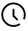剩余5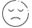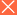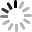乐清湾港区绿荫路建设工程（一期）-乐清湾港区绿荫路建设工程（一期） 下载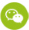扫描微信服务号•

中标结果打包下载

人工查询最新进展

发布招标采购信息

提示对手新动态

每日接收定制信息

供应商邀请参与招标

标书代写免费报价